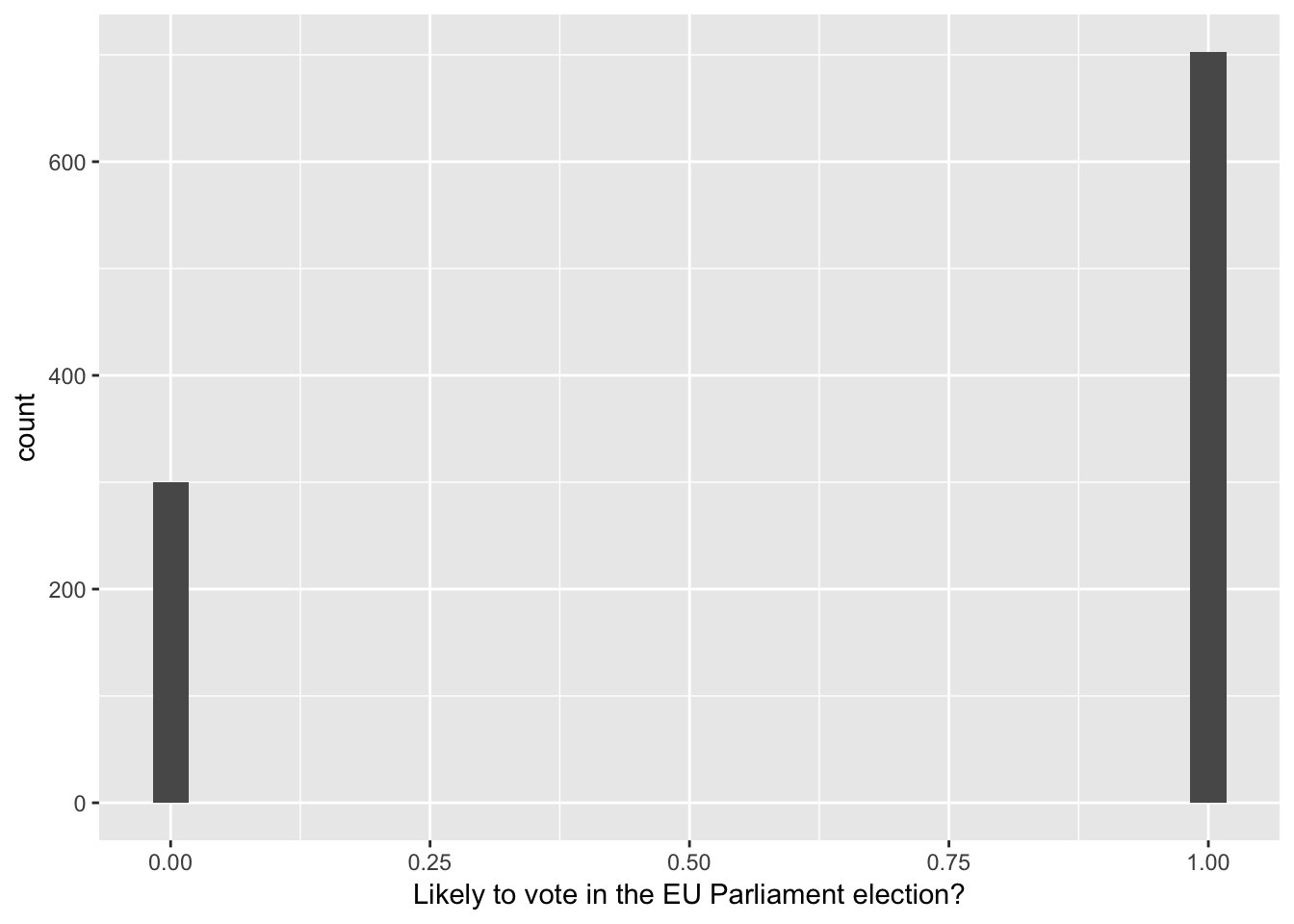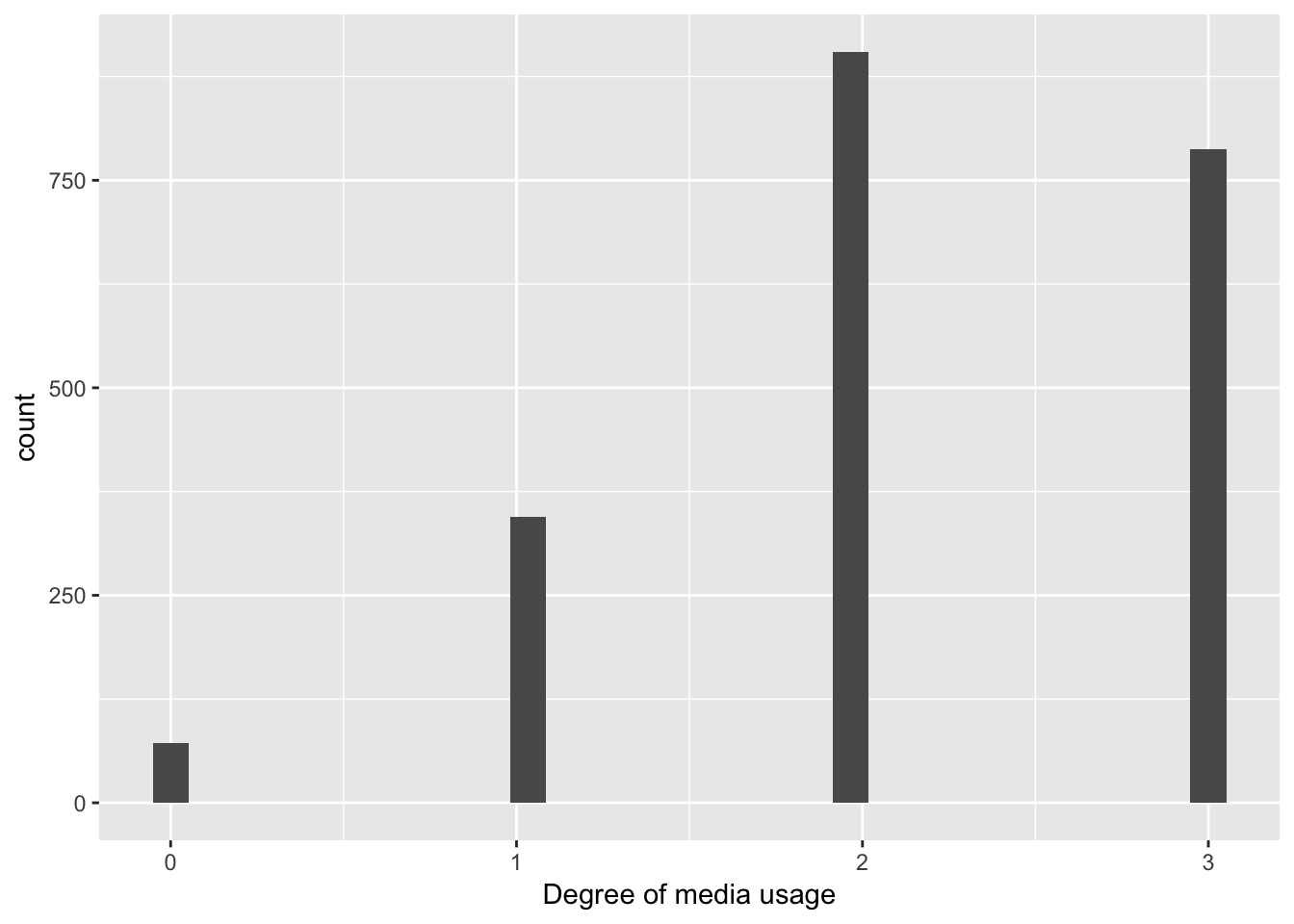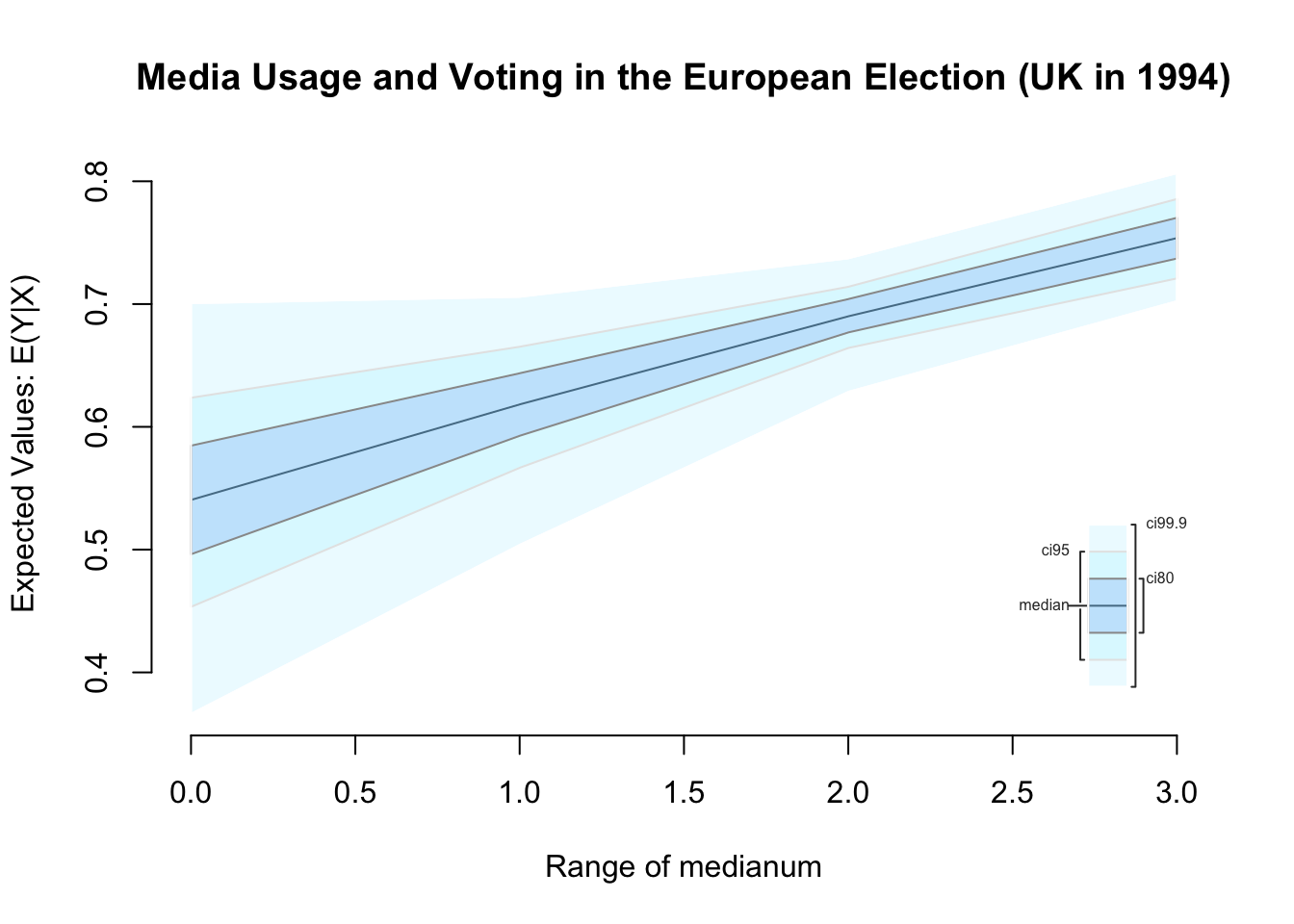# A quick-start guide to the statistical analysis of political attitudes and behaviors

Student resources

# Overview

The purpose of this document is to get you up and running with (potentially) professional-level, statistical analysis of political data, quickly. It will automatically import the Eurobarometer Trend File (1970-2002), a large survey dataset asking individuals across European countries a wide variety of questions in many different years. This document is intended to rapidly “bootstrap” individuals with zero programming knowledge into being able to conduct fairly sophisticated anlayses on a wide variety of political questions. In particular, this is intended especially for undergraduate political science students with no background in computer programming, interested in questions about political attitudes/behaviors, who wish to challenge themselves and build their skills/experience with quantitative data analysis. You can use this as a springboard to learning how to use R independently, but this document is not intended to teach you that. It’s a purely practical guide to jumpstart your capacity to produce analyses; everything else is up to you.

If you find this fun/interesting/useful/exciting, then you might want to actually learn the language a bit more. For excellent, easy, free interactive tutorials try: https://www.datacamp.com/courses/free-introduction-to-r and/or http://tryr.codeschool.com/

# Setup

4. Open RStudio

R is the programming language and RStudio is the application we will actually open, see, and do our work in.

1. Load this starter script I have written for you.

After opening RStudio, go to File > New File > R script and copy-paste that starter script into the new script file. Or download that that starter script as a .R file and then go to File > Open File and select that .R file. You can just select all of the text in that script and click “Run” (or command-Enter on a Mac), to rapidly have your computer do some basic data wrangling tasks and estimate, as well as visualize, the relationship between media exposure and the probability that individuals in the UK would vote in the 1994 European Parliament elections. To do your own original analysis of something totally different, you can peruse the codebook of the Eurobarometer data and copy/tinker with the code in my script to apply it to your own variables of interest. You can also choose different countries in different years, but in your first analyses, focus on one country in one year because things get statistically complicated when you have multiple countries and multiple years.

# Run the script and voilà

### Starter script for analyzing the Eurobarometer Trend data (1970-2002).
### Outline:
### 1. Import the dataset straight from the web
### 2. Isolate variables of interest and clean them up
### 3. Estimate a regression model of what makes people turnout for EP elections

### Remove the "#" in the following three lines if you have not already installed these
# install.packages("ggplot2")
# install.packages("foreign")
require(ggplot2)
require(foreign)
require(Zelig)

### Define the URL where the dataset is located
url<-"https://www.dropbox.com/s/5bdhel8l7c5r59z/eurobarometer_trends.dta?raw=1"

### Read the dataset straight from the web, name the dataframe "data"

### Variable "mediause" asks how much the respondent relies on the media.
summary(data$mediause) # Get a simple summary ## VERY HIGH high low VERY LOW dk na inap ## 166824 128310 71049 19534 2514 445 5612 ## NA's ## 740096 data$mediause[data$mediause=="dk"]<-NA # Let's consider all the "dk" answerers as missing (NA) data$mediause[data$mediause=="na"]<-NA # Let's consider all the "dk" as missing (NA) data$mediause[data$mediause=="inap"]<-NA # Let's consider all the "inap" as missing (NA) data$mediause<-factor(data$mediause) # this removes unused levels levels(data$mediause)<-c("Very high", "High", "Low", "Very low") # Clean up the messy names of the levels
data$medianum<-as.numeric(data$mediause) # convert the categories to a numerical scale (cheating a bit!)
data$medianum<-(4 - data$medianum) # Subtract from 4 to make it more intuitive (higher number = more likely to vote)

### Variable "particip" asks respondent how likely they are to vote in the EP elections.
summary(data$particip) ## CERTAINLY YES PROBABLY YES PROBABLY NOT CERTAINLY NOT depends ## 86318 37950 13719 16375 6192 ## DK,NA inap NA's ## 10030 7319 956481 data$particip[data$particip=="DK,NA"]<-NA data$particip[data$particip=="depends"]<-NA data$particip[data$particip=="inap"]<-NA data$particip<-factor(data$particip) levels(data$particip)<-c("Certainly yes", "Probably yes", "Probably not", "Certainly not")
data$participnum<-as.numeric(data$particip) # convert the categories to a numerical scale (cheating a bit!)
data$participnum<-(4 - data$participnum) # Subtract from 4 to make it more intuitive (higher number = more likely to vote)
data$particip <- ifelse(data$participnum>=2, 1, 0) # Collapse into a no/yes, 0/1, binary version

### Variable "income" asks the respondent's income level.
summary(data$income) ## Min. 1st Qu. Median Mean 3rd Qu. Max. NA's ## 1.00 5.00 8.00 29.28 96.00 99.00 52308 ### Variable "polint" asks the respondent's political interest generally. summary(data$polint)
##   A GREAT DEAL TO SOME EXTENT       NOT MUCH     NOT AT ALL          DK,NA
##          12311          36657          35622          24101            968
##           inap           NA's
##           2583        1022142
data$polint[data$polint=="DK,NA"]<-NA
data$polint[data$polint=="inap"]<-NA
data$polint<-factor(data$polint)
levels(data$polint)<-c("A great deal", "To some extent", "Not much", "Not at all") data$polintnum<-as.numeric(data$polint) # convert the categories to a numerical scale (cheating a bit!) data$polintnum<-(4 - data$polintnum) # Subtract from 4 to make it more intuitive (higher number = more likely to vote) summary(data$polintnum)
##    Min. 1st Qu.  Median    Mean 3rd Qu.    Max.    NA's
##     0.0     1.0     1.0     1.3     2.0     3.0 1025693
### Variable "ecint3" asks the respondent's interest in EU politics.
summary(data$ecint3) ## VERY INTERESTED A LITTLE NOT AT ALL DK, NA ## 17438 39654 20800 2727 ## inap NA's ## 0 1053765 data$ecint3[data$ecint3=="DK, NA"]<-NA data$ecint3[data$ecint3=="inap"]<-NA data$ecint3<-factor(data$ecint3) levels(data$ecint3)<-c("Very interested", "A little", "Not at all")
data$ecint3num<-as.numeric(data$ecint3) # convert the categories to a numerical scale (cheating a bit!)
data$ecint3num<-(3 - data$ecint3num) # Subtract from 4 to make it more intuitive (higher number = more likely to vote)
summary(data$ecint3num) ## Min. 1st Qu. Median Mean 3rd Qu. Max. NA's ## 0 0 1 1 1 2 1056492 ### Variable "ecint4" asks the respondent's interest in EU politics. summary(data$ecint4)
##   A GREAT DEAL TO SOME EXTENT       NOT MUCH     NOT AT ALL         DK, NA
##          14316          48580          49690          28588           2288
##           inap           NA's
##           4611         986311
data$ecint4[data$ecint4=="DK, NA"]<-NA
data$ecint4[data$ecint4=="inap"]<-NA
data$ecint4<-factor(data$ecint4)
levels(data$ecint4)<-c("A great deal", "To some extent", "Not much", "Not at all") data$ecint4num<-as.numeric(data$ecint4) # convert the categories to a numerical scale (cheating a bit!) data$ecint4num<-(4 - data$ecint4num) # Subtract from 4 to make it more intuitive (higher number = more likely to vote) summary(data$ecint4num)
##    Min. 1st Qu.  Median    Mean 3rd Qu.    Max.    NA's
##     0.0     1.0     1.0     1.3     2.0     3.0  993210
### Variable "nation1" captures the respondent's nation
summary(data$nation1) ## france belgium netherlands GERMANY-WEST ## 91414 89906 89269 91498 ## italy luxembourg denmark ireland ## 92163 38459 84566 84395 ## GREAT BRITAIN NORTHERN IRELAND greece spain ## 88792 25303 70266 60123 ## portugal GERMANY-EAST norway finland ## 59947 49641 11989 37869 ## sweden austria switzerland ## 33671 35113 0 data$gb<-ifelse(data$nation1=="GREAT BRITAIN", 1, 0) ### Variable "year" captures the year of the survey summary(data$year)
##    Min. 1st Qu.  Median    Mean 3rd Qu.    Max.
##    1970    1987    1994    1992    1999    2002
##########################################################################
### Zero in on the subset of the sample relevant to your research question
##########################################################################

# Subset the data to one country in one year because having multiple countries/years gets complicated statistically
dataUK1994<-subset(data, nation1=="GREAT BRITAIN" & data$year==1994, select=c("particip", "medianum", "polintnum", "income", "nation1")) ######################################################################### ### Always do some basic visual inspection / descriptive analysis of your key variables qplot(dataUK1994$particip) +
labs(x="Likely to vote in the EU Parliament election?")qplot(dataUK1994$medianum) + labs(x="Degree of media usage")####################################################### ####### Regression analysis using the Zelig package ####################################################### # DV is likely to vote or not likely to vote, i.e. a binary variable. # So we need a "logit" model model<-zelig(particip ~ income + medianum, data=dataUK1994, model="logit") ## How to cite this model in Zelig: ## R Core Team. 2007. ## logit: Logistic Regression for Dichotomous Dependent Variables ## in Christine Choirat, James Honaker, Kosuke Imai, Gary King, and Olivia Lau, ## "Zelig: Everyone's Statistical Software," http://zeligproject.org/ summary(model) ## Model: ## ## Call: ## z5$zelig(formula = particip ~ income + medianum, data = dataUK1994)
##
## Deviance Residuals:
##     Min       1Q   Median       3Q      Max
## -1.7077  -1.4148   0.7351   0.8428   1.1639
##
## Coefficients:
##              Estimate Std. Error z value Pr(>|z|)
## (Intercept)  0.234961   0.211419   1.111 0.266418
## income      -0.002073   0.001648  -1.257 0.208654
## medianum     0.320133   0.087705   3.650 0.000262
##
## (Dispersion parameter for binomial family taken to be 1)
##
##     Null deviance: 1220.7  on 1000  degrees of freedom
## Residual deviance: 1205.5  on  998  degrees of freedom
##   (2189 observations deleted due to missingness)
## AIC: 1211.5
##
## Number of Fisher Scoring iterations: 4
##
## Next step: Use 'setx' method
x.out <- setx(model, medianum=seq(0,3,1)) # Set media to a range from its min to its max
s.out <- sim(model, x = x.out)

ci.plot(s.out,
main="Media Usage and Voting in the European Election (UK in 1994)")